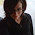### Equivalent fractions worksheets with pictures

A recent addition to my site Homeschoolmath.net... free worksheets for equivalent fractions with pie images (or not... they're optional).

There are five problem types to choose from:
1. Two fractions are given with 2 empty pie images to color in (e.g. 3/5 = 6/10).
2. There are 2 pie images that are already colored; the student writes both fractions.
3. Two pie images are given, one colored in, one not; the student writes both fractions.
4. There are 2 pie images to color, one fraction is given, one not (e.g. 4/5 =    /    )
5. Problems without any visual model; the student writes the missing numerator or denominator in one of the fractions (e.g. 2/3 =    /12).

Check them out! These are meant for grades 3 and 4 in the Common Core standards.Beth said…
Thanks for sharing. I just found your blog and you have a ton of great information and resources :)This looks like a great tool to use for creating equivalent fraction worksheets. Thanks!